Courses

# Analytic Functions (Part - 1)- Mathematical Methods of Physics, UGC - NET Physics Physics Notes | EduRev

## Physics for IIT JAM, UGC - NET, CSIR NET

Created by: Akhilesh Thakur

## Physics : Analytic Functions (Part - 1)- Mathematical Methods of Physics, UGC - NET Physics Physics Notes | EduRev

The document Analytic Functions (Part - 1)- Mathematical Methods of Physics, UGC - NET Physics Physics Notes | EduRev is a part of the Physics Course Physics for IIT JAM, UGC - NET, CSIR NET.
All you need of Physics at this link: Physics

1. Some Properties of Analytic Functions :  We begin by reviewing briefly some fundamental points in the theory of analytic functions in a form which will be convenient for further references. Departing slightly from customary notations, we shall write w = v+iu, and we shall consider the theory primarily as the theory of a system of differential equations

(1)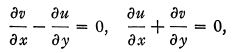which are called the Cauchy-Riemann equations. We shall not enter into various fine points which arise in the discussion, but we may mention that the functions u and v, as well as the other functions which appear later, must be assumed to be differentiable, that is, to possess complete differentials in the sense of Stolz.

(a) One point of view often taken in applications is that we have a vector or, rather a vector field, of components (u} v), and that the differential equations express the fact that the rotation (Curl) and the divergence of this vector are zero.

Another point of view, which we shall find extremely useful, is that we have two vectors ƒ and r, whose components are f1 = u, f2 = v, and n = v, r2= —u, respectively, and that the differential equations express the fact that the divergences of both are zero. These two vectors, as the relations

(2) f1 = - r2, f2 = r1

show, are perpendicular and of equal length, so that we may say that the theory of analytic functions is the theory of two equal and perpendicular vectors in the plane, with zero divergences.

(b) The differential equations may be considered as integrability conditions. They are equivalent to the vanishing of certain contour integrals, taken around contours inside of which the functions are regular.

(c) If the functions are not regular inside a contour but have singularities in one point, the integrals do not in general vanish, but furnish two real numbers (which taken together as a complex number form a residue) and which characterize to a certain extent the singularity. The simplest type of singularity is shown by the function w = ci/z, for which in our notations we have

(3)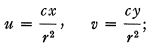the residue in this case is equal to ci. (Here c is a real number and r= x2+y2.)

(d) By elimination of one of the functions (w, v), we obtain for the other the second-order (Laplace) equation

(4)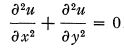(e) There are certain second-degree quantities that might be discussed in connection with an analytic function ; these are

(5)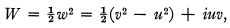half the square of the function ; and

(6)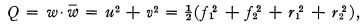the square of the modulus, or the norm of the function. By the fact that (u, v) satisfy the differential equations (1) certain conditions are imposed on these second-degree quantities, and we shall now write them down.

For W it is easy ; the statement that w is an analytic function means the same as the statement that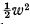is an analytic function, and the latter statement is equivalent to the relations

(7)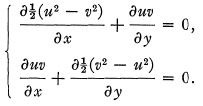This system of differential equations is then equivalent to system (1), and it is easy to verify this fact directly.

The situation is more complicated with respect to Q. The easiest way to arrive at the differential equation which must be satisfied by Q as a result of the fact that u> v satisfy (1) seems to be the consideration of log w2. This must be analytic if w is, and therefore the real part of log w2 must satisfy the Laplace equation. But the real part of log w2 is log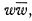, so that we have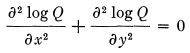or

(8)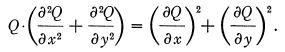It is interesting to note that this equation is non-linear, and that it therefore gives an example of a non-linear equation which is a consequence of a system of linear equations. We next ask ourselves whether the condition expressed by the last equation is sufficient, in other words whether every function Q satisfying this condition may be considered as the norm of an analytic function.

In the first place it is clear that a function is not entirely determined by its norm. If the function w = v+iu has the norm (?, any function V+iU% for which

(9)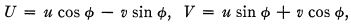where φ is an arbitrary function of x and y, will have the same norm. The question then reduces to this: are there among the functions V+iU some that are analytic? An easy calculation leads to the result that the equations

(10)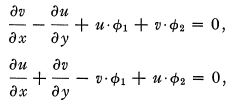with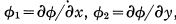must be satisfied. Further calculation shows that the integrability condition for these equations is exactly the above equation (7) for Q.

(f) An analytic function can be developed into a power series. In view of an analogy that we want to establish later the following way to introduce this series might find its place here. We first write the Cauchy-Riemann equations (1) in the form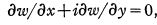and, passing to polar coordinates, we have

(11)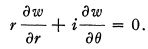Next, making use of the fact that the equation does not contain 6 explicitly, and therefore must allow solutions of the form
(12)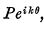where P is independent of 0, we find for P the equation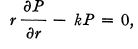which has a solution P = rk, so that

(13)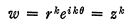is a solution of the original equation. If we require the solution to be one-valued, k must be an integer, and if we want it to be continuous (at the origin), k must be non-negative. A linear combination of a finite number of such solutions is a polynomial, and the general solution may be presented as a linear combination of an infinite number of such solutions which we may consider as the limit of a (uniformly convergent) sequence of polynomials, or, if you prefer, as a power series.

2. The Volterra Theory. : We pass now to generalizations. We saw that the theory of analytic functions may be considered as the theory of two equal and perpendicular vectors with vanishing divergences. We may try to extend this to the three-dimensional space. If we take two vectors ƒ and r and write down the conditions for their equality and perpendicularity, we find

(14)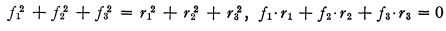and although the differential equations

(15)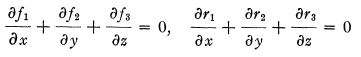are linear, the system as a whole is not. The same is true in the two-dimensional case, of course, but there the equations are reduced to linear equations easily. We shall not consider now the question whether these equations can be reduced to linear equations  ; trying to keep more or less to the historical order we shall outline a way out from this difficulty, which leads to Volterra's theory of conjugate functions dated back to 1889. We may consider a vector as a finite portion of a directed line (or curve), and its components as the lengths of the projections of this finite portion on the coordinate axes. If now we consider a finite portion of a plane, or surface, as a surface vector, and as its components the areas of its projections on the coordinate planes, we have a new object to operate upon. The general case may be reduced to that of a triangle with vertices at (0, 0, 0), (x1, x2, X3), (y1, y2, y3). The areas of the projections are the determinants of the matrix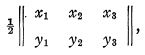which we may denote by R23, R31 , R12 or L , M, N, or in general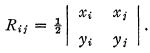It may seem sufficient to consider only the above three components; however, it is more convenient not to restrict i and j , bu t to use all nine combinations, introducing th e relation Xij+Xji = 0. These nine may be arranged into a square matrix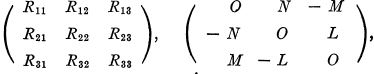(16)

so that a surface vector is represented by a matrix possessing the property of antisymmetry. Consider now a line vector X = F1, Y=F2. Z — F3 with the above surface vector. Conditions of perpendicularity and numerical equality may be shown to result simply in the equations

(17 )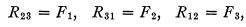which should be compared with the equations (2). If now we impose the vanishing of divergences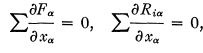and write out everything without indices in terms of X, Y, Z, x, y, z} we obtain a system of four equations

(18)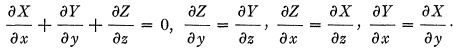This is an analog for three-space of the Cauchy-Riemann system. It is worth noting that setting Z = 0 we get two functions X and Y depending on x and y alone which satisfy the equations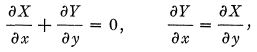that is, exactly the Cauchy-Riemann equations for X = u, Y = v. We further note that the elimination of two of the three functions X, F, Z from the above four equations leads as in (d),  to the Laplace equation in three dimensions. We have thus a theory in three-space which may be considered as a generalization of the theory of analytic functions, and which is essentially the theory of two equal and perpendicular vectors with vanishing divergences, one of these vectors being a line vector, and the other a surface vector.

There are no essential difficulties (except that of losing the help of intuition) in extending the theory to any number of dimensions. We shall only take up the case n = 4. Here we may consider the case of two perpendicular surface vectors with vanishing divergences. There are here six coordinate planes, and therefore every surface vector has six components. They are known as six-vectors. We may write, using notations similar to those used above, fij for the components, with the condition fij + fji = 0. In four-space, this will mean a square four-rowed matrix, whose sixteen elements reduce to six as a result of these relations. The conditions of numerical equality and perpendicu-larity for two such vectors ƒ and r again reduce to linear relations, namely,

(19)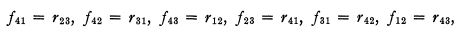which should be compared with (2) and (18). The divergence equations together with these relations constitute a linear system which is analogous to, and a generalization of, the CauchyRiemann system (1). This system may be written as

(20)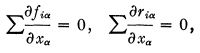or, in full, if we set

(21)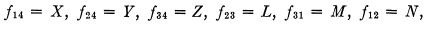(22)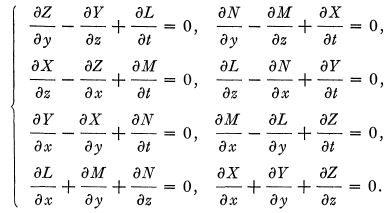Here again the elimination of all but one component leads to a Laplace equation. As I have said, analogous considerations apply to spaces of any number of dimensions, and when the sum of the numbers of dimensions of the two vectors is equal to the number of dimensions of the space the system reduces to a linear system—this is the case studied by Volterra.  Volterra's work has been followed up by Lagally, de Donder, Dixon,f and a few others, but very much remains to be done. Many features of the theory of analytic functions are preserved, but not all. Complex numbers or hypercomplex numbers are not used except in the four-dimensional case by Dixon. The three-dimensional theory bears the same relationship to the Newtonian potential as the theory of analytic functions to the logarithmic potential, and some results of potential theory can be directly translated into this theory, but there are some questions which have to be treated independently. Of course, the differential equations may be replaced by integral conditions, by the vanishing of certain integrals taken over surfaces surrounding volumes in which the functions are regular. An extension of the Cauchy integral formula can be proved for all cases. There are different types of singularities, point singularities, line singularities, etc. The theory of residues presents a particular fascination. Expansions analogous to power series exist; in the three-dimensional case they are essentially developments into series of harmonic functions. But we shall abandon now the purely mathematical developments and pass to applications.

Offer running on EduRev: Apply code STAYHOME200 to get INR 200 off on our premium plan EduRev Infinity!

159 docs

,

,

,

,

,

,

,

,

,

,

,

,

,

,

,

,

,

,

,

,

,

,

,

,

;